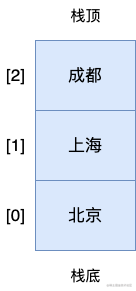# 数组实现栈

## 什么是栈？## 实现一个栈

`class Stack {  constructor() {    this.lists = [];  }}`

• `push()`：添加新元素到栈顶
• `pop()`：移除栈顶底新元素
• `peek()`：返回栈顶底元素
• `isEmpty()`：判断栈里是否有元素，没有则返回 true
• `clear()`：清除栈里的所有元素
• `size()`：返回栈里元素的数量

`class Stack {  constructor() {    this.lists = [];  }  // 入栈  push(item) {    this.lists.push(item);  }  // 出栈  pop() {    this.lists.pop();  }  peek() {    this.lists[this.lists.length - 1];  }  isEmpty() {    return this.lists.length == 0;  }  clear() {    this.lists = [];  }  size() {    return this.lists.length;  }}`

`var stack = new Stack();console.log(stack.isEmpty()); // true`

`stack.push('北京');stack.push('上海');console.log(stack.size()); // 2console.log(stack.peek()); // '上海'`

`stack.push('成都');console.log(stack.size()); // 3console.log(stack.peek()); // '成都'console.log(stack.lists); // ['北京', '上海', '成都']`

`stack.pop();console.log(stack.size()); // 2console.log(stack.lists); // ['北京', '上海']`